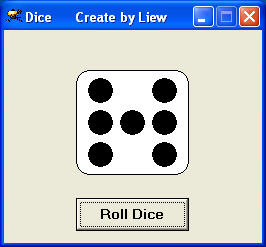# Animated Dice

This program is similar to the dice program except that we have added animation to the program. To add the animated effect, you need to include the timer control into your program. In the timer's properties windows, set the timer enabled property to false and the  interval to 10. You can change the interval to any number you want. Insert a command button and change its label to Roll_Dice. Click on it and set the timer to enabled using the statement Timer1.Enabled = True.

You also need to create a subroutine and name it as roll. Under the roll subroutine, enter the code that display the different faces of the dice. To create the animated effect,  you need to declare a global variable x and assign an initial value of 0 to it, then include the statement  x=x+10 so that the value of x increase 10 units after an interval of 10 milliseconds. Under the timer subroutine, use the If ..then statement to stop the timer when x>1000. Now, when you click the button roll dice, you will see that faces of the dice changes and then come to a stop### The Code

Dim x As Integer

Sub roll( )
x = x + 10
Randomize Timer
n = Int(1 + Rnd * 6)
For i = 0 To 6
Shape1(i).Visible = False
Next
If n = 1 Then
Shape1(3).Visible = True
Shape2.FillColor = &HC0C0C0

End If
If n = 2 Then
Shape1(2).Visible = True
Shape1(4).Visible = True
Shape2.FillColor = &H8080FF
End If
If n = 3 Then
Shape1(2).Visible = True
Shape1(3).Visible = True
Shape1(4).Visible = True
Shape2.FillColor = &H80FF&
End If
If n = 4 Then
Shape1(0).Visible = True
Shape1(2).Visible = True
Shape1(4).Visible = True
Shape1(6).Visible = True
Shape2.FillColor = &HFFFF00
End If

If n = 5 Then
Shape1(0).Visible = True
Shape1(2).Visible = True
Shape1(3).Visible = True
Shape1(4).Visible = True
Shape1(6).Visible = True
Shape2.FillColor = &HFFFF&
End If
If n = 6 Then
Shape1(0).Visible = True
Shape1(1).Visible = True
Shape1(2).Visible = True
Shape1(4).Visible = True
Shape1(5).Visible = True
Shape1(6).Visible = True
Shape2.FillColor = &HFF00FF
End If
End Sub

Private Sub Roll_Dice_Click()
Timer1.Enabled = True
x = 0
End Sub

Private Sub Timer1_Timer()
If x < 1000 Then
roll
Else
Timer1.Enabled = False
End If
End Sub